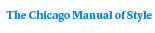# Inversions

I. Ya. Bakel'manTranslated by Joan W. Teller and Susan Williams
77 pages | 6 x 9 | © 1974
Paper \$28.00 ISBN: 9780226034997 Published February 1975
In this book, I. Ya. Bakel’man introduces inversion transformations in the Euclidean plane and discusses the interrelationships among more general mathematical concepts. The author begins by defining and giving examples of the concept of a transformation in the Euclidean plane, and then explains the "point of infinity" and the "stereographic projection" of the sphere onto the plane. With this preparation, the student is capable of applying the theory of inversions to classical construction problems in the plane.

The author also discusses the theory of pencils of circles, and he uses the acquired techniques in a proof of Ptolemy’s theorem. In the final chapter, the idea of a group is introduced with applications of group theory to geometry. The author demonstrates the group-theoretic basis for the distinction between Euclidean and Lobachevskian geometry.